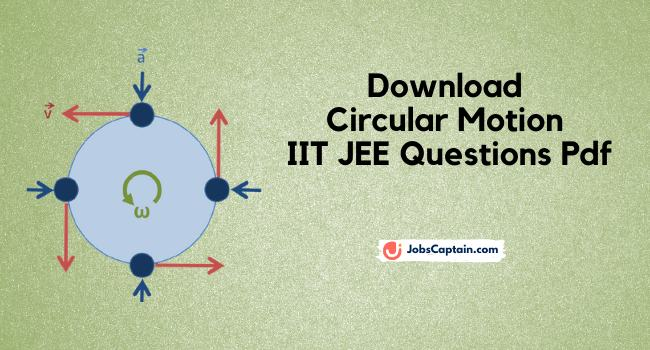# Circular Motion IIT JEE Questions PDF Notes

By | March 19, 2021In physics, circular motion is an object moving along the circumference of a circle or rotation along a circular path. Question bank on circular motion pdf is prepared by expert teachers so that students can easily understand it.

Circular motion IIT JEE notes pdf contains objective questions (MCQ), subjective questions, comprehension and statement matching problems, previous years asked questions of IIT, JEE Mains and Advanced exams.

Circular motion questions and answers pdf provides answer keys and solutions to all the problems with detailed explanations. Circular motion NEET questions pdf gives various circular motion examples for practice.

It covers many circular motion questions that are asked in IIT and JEE exams.  You can download this circular motion solved pdf from here.

Circular motion is classified into two types:

Uniform Circular Motion

Non-uniform Circular Motion